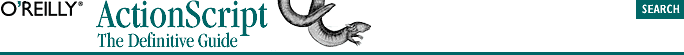home | O'Reilly's CD bookshelfs | FreeBSD | Linux | Cisco | Cisco Exam## 9.9. Recursive Functions

```function trouble( ) {
trouble( );
}```

Practical recursive functions call themselves only while a given condition is met (thus preventing infinite recursion). Example 9-4 used recursion to count from a specified number down to 1, but obviously that can be accomplished without recursion.

One classic use of recursion is to calculate the mathematical factorial of a number. The factorial of 3 (written as 3! in mathematical nomenclature) is 3*2*1=6. The factorial of 5 is 5*4*3*2*1=120. Example 9-8 shows a factorial function that uses recursion.

#### Example 9-8. Calculating Factorials Using Recursion

```function factorial(x) {
if (x < 0) {
return undefined;  // Error condition
} else if (x <= 1) {
return 1;
} else {
return x * factorial(x-1);
}
}
trace (factorial(3));  // Displays: 6
trace (factorial(5));  // Displays: 120```

#### Example 9-9. Calculating Factorials Without Recursion

```function factorial(x) {
if (x < 0) {
return undefined; // Error condition
} else {
var result = 1;
for (var i = 1; i <= x; i++) {
result = result * i;
}
return result;
}
}```

Example 9-8 and Example 9-9 represent two ways of solving the same problem. The recursive method says, "The factorial of 6 is 6 multiplied by the factorial of 5. The factorial of 5 is 5 multiplied by the factorial of 4 . . . " and so on. The nonrecursive method loops over the numbers from 1 to x and multiplies them all together into one big number.

Which approach is better -- recursive or nonrecursive -- depends on the problem. Some problems are solved more easily using recursion, but recursion can be slower than nonrecursive solutions. Recursion is best used when you don't know how deeply a data structure may be nested. For example, suppose you wanted to list all the files within a subdirectory, including listing all files within any nested subdirectory, ad infinitum. You couldn't write a general solution that worked for any number of subdirectories without resorting to recursion. A recursive solution might look like this in pseudocode:

```function listFiles (directoryName) {
do (check the next item in directoryName) {
if (this item is a subDirectory itself) {
// Recursively call this function with the new subdirectory
listFiles(subDirectoryName);
} else {
// Display the name of this file
trace (filename);
}
} while (there are still items to check);
}```

When we consider the XML object in Part III, "Language Reference", we'll use recursion to list all the elements in an XML document.